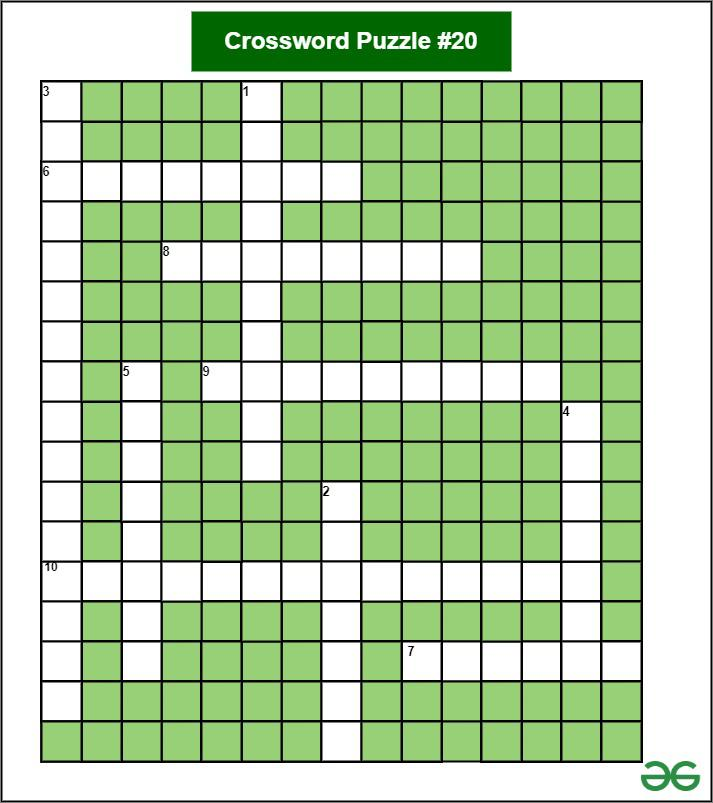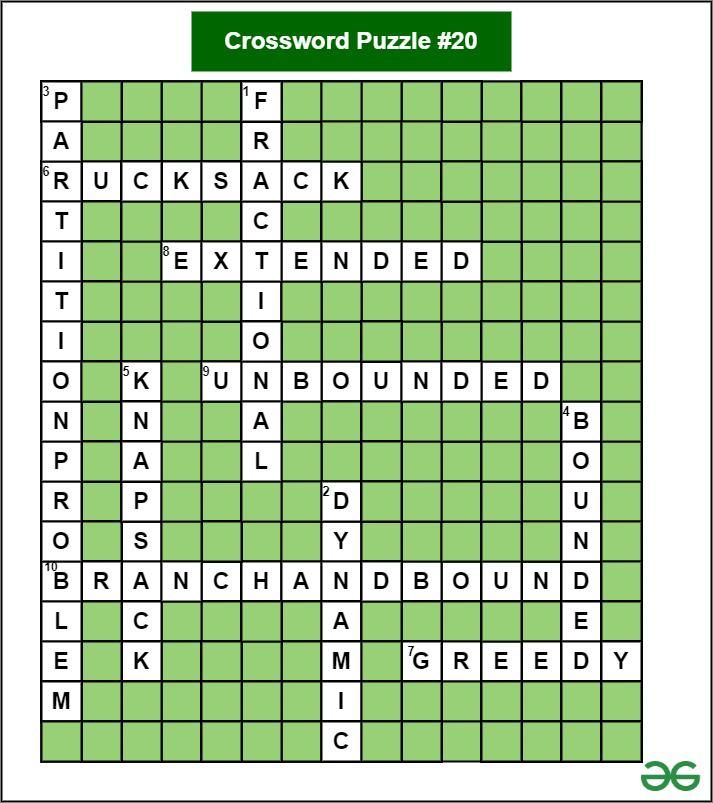GFG App
Open AppBrowser
Continue

# Crossword Puzzle Of The Week #20 (KnapSack Problem)

In this issue of  Crossword Puzzle of the Week, we will dive into the topic of the Knapsack ProblemThe solution to the crossword puzzle is provided at the end.### HINTS:

Across:

1. In _____ Knapsack, we can break items for maximizing the total value of the knapsack.

2. 0/1 Knapsack problem has both properties (Overlapping Subproblem and Optimal Substructure Property) of a _____ programming problem.

3. The _____ is a classical Knapsack used to determine whether a given set can be partitioned into two subsets such that the sum of elements in both subsets is the same.

4. The _____ Knapsack Problem is a generalization of the 0-1 Knapsack Problem where a bounded amount of each item type is available

5. Given a bag with maximum weight capacity of W and a set of items, each having a weight and a value associated with it. Decide the number of each item to take in a collection such that the total weight is less than the capacity and the total value is maximized. The Problem is _____ Problem.

Down:

6. The Knapsack problem is an example of the combinational optimization problem. This problem is also commonly known as the _____ Problem.

7. The Fractional Knapsack problem uses _____ approach.

8. _____ Problem is a variation of Bounded Knapsack Problem in which Given items, each item having a given weight Ci and a profit value Pi, the task is to maximize the profit by selecting a maximum of K items adding up to a maximum weight W.

9. _____ Knapsack is different from classical Knapsack problem, here we are allowed to use unlimited number of instances of an item.

10. _____ is an algorithm design paradigm which is generally used for solving combinatorial optimization problems.

## Solution for Crossword Puzzle #20:Down:

2. DYNAMIC

4. BOUNDED

5. KNAPSACK

Across:

6. GREEDY

8. EXTENDED

My Personal Notes arrow_drop_up